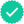#### Related questions#### Concept explainers

Question

Need answer to the following question.

Expert SolutionStep 1

We first find the slope of the tangent for which we need derivative of f(x).  Differentiating f(x) w.r.t. x we get:

Step 2

Now, slope is the value of f’(x) at the given point x = 1.

Step 3

Now, to find any one point on the tangent line, we consider the fact that tangent line is drawn at x=1. This point also lies on the curve and hence its y-coordinate is the value of f(x) at x=1. We get:Trending nowThis is a popular solution!Step by stepSolved in 5 steps with 5 imagesKnowledge BoosterRecommended textbooks for you
•Trigonometry (MindTap Course List)
Trigonometry
ISBN:9781337278461
Author:Ron Larson
Publisher:Cengage LearningCollege Algebra
Algebra
ISBN:9781337282291
Author:Ron Larson
Publisher:Cengage Learning
•Trigonometry (MindTap Course List)
Trigonometry
ISBN:9781337278461
Author:Ron Larson
Publisher:Cengage LearningCollege Algebra
Algebra
ISBN:9781337282291
Author:Ron Larson
Publisher:Cengage Learning